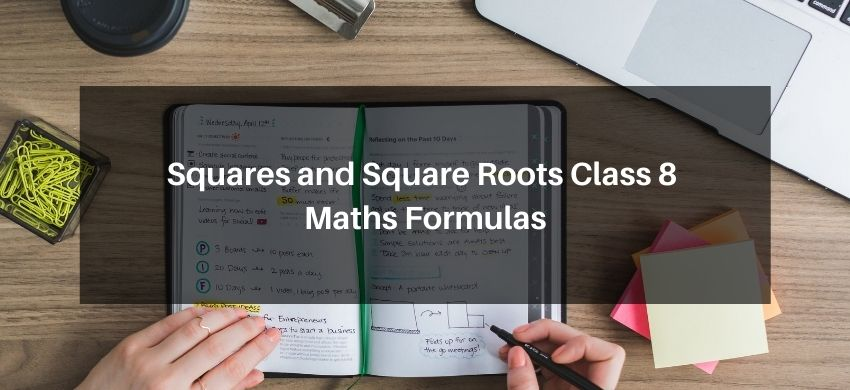Most Affordable JEE | NEET | 8,9,10 Preparation by Kota's Top IITian Doctor Faculties

# Squares and Square Roots Class 8 Maths FormulasHey, students are you looking for Squares and Square Roots Class 8 Maths Formulas? If yes. Then you are at the right place. In this post, I have listed all the formulas of Squares and Square Roots class 8 that you can use to learn and understand the concepts easily.

If you want to improve your class 8 Math, Squares and Square Roots concepts, then it is super important for you to learn and understand all the formulas.

By using these formulas you will learn about Squares and Square Roots.

With the help of these formulas, you can revise the entire chapter easily.

## Squares and Square Roots Class 8 Maths Formulas

Square Number: The square of a number is the product of the number with the number itself The, square of x = (x × x), denoted by x 2.

A natural number n is a perfect square, if n = m2 for some natural number m.{1 = 1 × 1 = 12, 4 = 2 × 2 = 22}

Square Root: Square root is the inverse operation of square, i.e., positive square root of a number is denoted by the symbol $\sqrt{ }$

For example, $3^{2}=9$ gives $\sqrt{9}=3$ or $\left(3^{2}\right)^{1 / 2}=3 .$

For positive numbers $a$ and $b$, we have

(i) $\sqrt{a b}=\sqrt{a} \times \sqrt{b}$,

(ii) $\sqrt{\frac{a}{b}}=\frac{\sqrt{a}}{\sqrt{b}}$ or $\sqrt{\frac{a}{b}}=\left(\frac{a}{b}\right)^{\frac{1}{2}}$

### Properties of the Square Number:

• A number ending in 2, 3, 7 or 8 is never a perfect square.
• A number ending in an odd number of zeros is never a perfect square.
• The square of an even number is even.
• The square of an odd number is odd.
• The square of a proper fraction is smaller than the fraction.
• For every natural number $n$, we have $\left\{(n+1)^{2}-n^{2}\right\}=\{(n+1)+n\}$.
• Sum of first $n$ odd natural numbers $=n^{2}$.
• If $m, n, p$ are natural numbers such that $\left(m^{2}+n^{2}\right)=p^{2}$, then $(m, n, p)$ is called a Pythagorean triplet.
• For every natural number $m>1,\left(2 m, m^{2}-1, m^{2}+1\right)$ is a Pythagorean triplet.
• There are $2 n$ non-perfect square numbers between the squares of the number $n$ and $(n+1)$
• The numbers which can be expressed as the product of the number with itself are called square numbers or perfect squares. For example, $1,4,9,16,25, \ldots .$
• If a natural number $m$ can be expressed as $n^{2}$, where $n$ is also a natural number, then, $m$ is called a square number.

If you have any Confusion related to Squares and Square Roots Class 8 Maths Formulas, then feel free to ask in the comments section down below.

To watch Free Learning Videos on Class 8 Maths by Kota’s top Faculties Install the eSaral App